﻿ 基于微观组织结构重构的先进高强度双相钢力学性能预测

# 基于微观组织结构重构的先进高强度双相钢力学性能预测Prediction of the Mechanical Properties for Advanced High-Strength Dual Phase Steel Based on the Reconstructed Microstructure

Abstract: The two-point correlation and linear-path probability functions were used to statistically analyze and characterize the metallography of advanced high-strength dual phase steel observed by the optical microscope. Combined with simulated annealing optimization algorithm, a reconstruction method for the microstructure of dual phase steel was established. The RVE model was constructed based on the reconstructed microstructure. The tensile and shear mechanical properties were predicted by using the RVE model. The results showed that the typical microstructure of the dual phase steel with statistical significance can be obtained by combining the probability function with optimization algorithm. The mechanical properties predicted by RVE model base on the reconstructed microstructure are in good agreement with the experimental results.

1. 引言

2. 双相钢微观组织结构测试与表征

2.1. 材料与试样制备Table 1. Chemical composition of DP600Table 2. Basic mechanical properties of DP600

2.2. 微观组织结构表征

$O\left(p\right)=\left\{\begin{array}{cc}1& I\left(p\right)>T\\ 0& I\left(p\right)\le T\end{array}$ (1)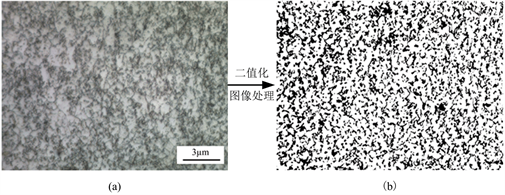Figure 1. Microstructure of DP600: (a) experimental measured metallography; (b) binarized image

${f}_{2}\left({p}_{1},{p}_{2}\right)=E\left[O\left({p}_{1}\right)O\left({p}_{2}\right)\right]$ (2)

${f}_{2}\left(r\right)=E\left[O\left({p}_{1}\right)O\left({p}_{2}\right)\right]$ (3)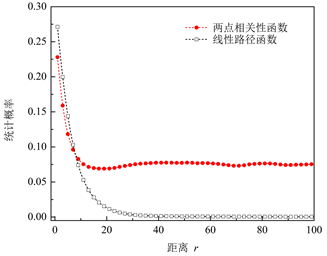Figure 2. Two-point correlation and linear-path probability function curve for microstructure of DP600

3. 双相钢微观组织结构重构

3.1. 重构模型与算法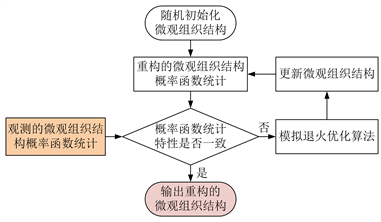Figure 3. The flowchart for DP600 microstructure reconstruction based on the simulated annealing algorithm

3.2. 重构结果与分析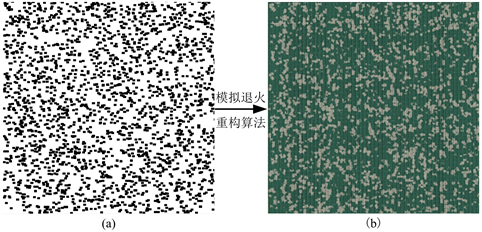Figure 4. The microstructure reconstruction for DP600: (a) initial result; (b) final result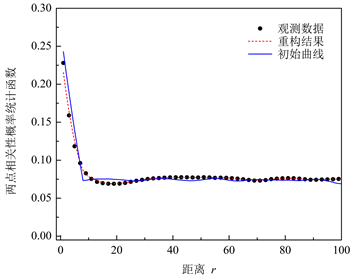Figure 5. Comparison of probability function curves between reconstructed DP600 microstructure and experimental observed metallography

4. 力学性能预测与分析

4.1. 代表体积元模型构建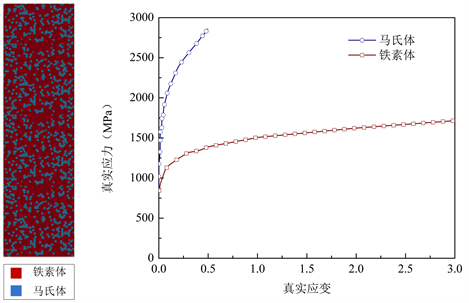Figure 6. The RVE model for mechanical property prediction of dual phase steel based on the reconstructed microstructure

4.2. 预测结果与验证

4.2.1 . 单向拉伸力学性能预测与验证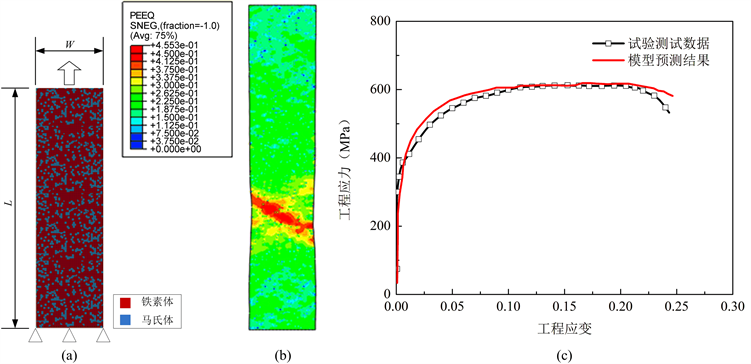Figure 7. The uniaxial tensile mechanical property prediction of DP600: (a) FE model; (b) Distribution of equivalent plastic strain; (c) Comparison of the stress-strain curve

4.2.2 . 剪切力学性能预测与验证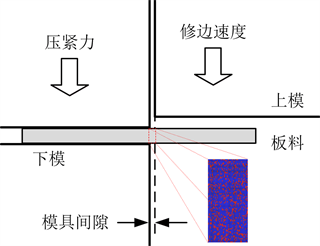Figure 8. The model for shear mechanical property prediction of DP600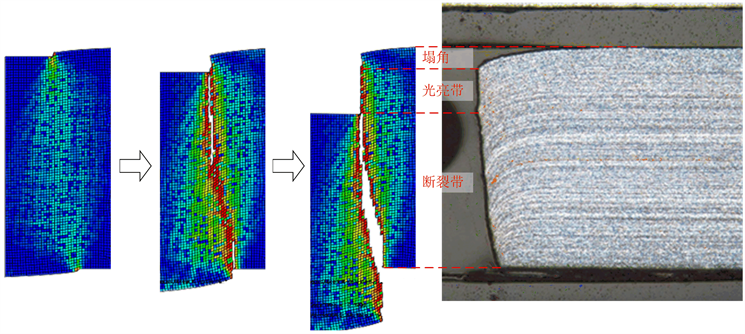Figure 9. The predicted result of shear mechanical property for DP600

5. 结论

1) 应用概率统计函数能较好的对双相钢的微观组织结构的相组分和形貌进行表征，结合优化算法，能重构得到双相钢具有统计意义的典型微观组织结构；

2) 基于重构的双相钢微观组织结构建立的代表体积元模型能得到与拉伸和剪切试验较为吻合的力学性能预测结果。

 Olson, G.B. (1997) Computational Design of Hierarchically Structured Materials. Science, 277, 1237-1242.
https://doi.org/10.1126/science.277.5330.1237

 Bhadeshia, H.K.D.H. and Honeycombe, R.W.K. (2006) Steels Microstructure and Properties. 3rd Edition, Elsevier Ltd., Amsterdam.
https://doi.org/10.1016/B978-075068084-4/50016-9

 Boudeau, N., Gelin, J. and Salhi, S. (1998) Computa-tional Prediction of the Localized Necking in Sheet Forming Based on Microstructural Material Aspects. Computational Materials Science, 11, 45-64.
https://doi.org/10.1016/S0927-0256(97)00153-5

 Sodjit, S. and Uthaisangsuk, V. (2012) Microstructure Based Prediction of Strain Hardening Behaviour of Dual Phase Steels. Materials & Design, 41, 370-379.
https://doi.org/10.1016/j.matdes.2012.05.010

 Paul, S.K. and Kumar, A. (2012) Micromechanics Based Modeling to Predict Flow Behavior and Plastic Strain Localization of Dual Phase Steels. Computational Materials Science, 63, 66-74.
https://doi.org/10.1016/j.commatsci.2012.05.061

 Yeong, C. and Torquato, S. (1998) Reconstructing Random Media. Physical Review E, 57, 495-506.
https://doi.org/10.1103/PhysRevE.57.495

 Jiao, Y., Stillinger, F. and Torquato, S. (2007) Modeling Hetero-geneous Materials via Two-Point Correlation Functions: Basic Principles. Physical Review E, 76, Article ID: 031110.
https://doi.org/10.1103/PhysRevE.76.031110

 李英. 镁合金微观结构的三维重构和有限元分析[D]: [硕士学位论文]. 青岛: 青岛科技大学, 2015.

 Liu, Y., Steven, G.M., Chen, W., Dikin, D.A. and Liu, W.K. (2013) Computational Microstructure Characterization and Reconstruction for Stochastic Multiscale Material Design. Com-puter-Aided Design, 45, 65-76.
https://doi.org/10.1016/j.cad.2012.03.007

 李奎荣. 钛合金微观组织结构计算机重构技术研究[D]: [硕士学位论文]. 青岛: 青岛科技大学, 2013.

 李奎荣, 王玉玲, 杨勇, 李明. 钛合金二维微观组织结构的计算机重构技术研究[J]. 机械科学与技术, 2015, 34(3): 485-488.

 杨志刚. 多晶体材料微观组织结构的计算机重构[D]: [硕士学位论文]. 兰州: 兰州理工大学, 2008.

Top